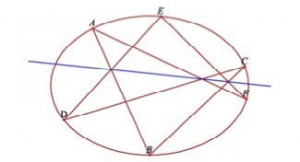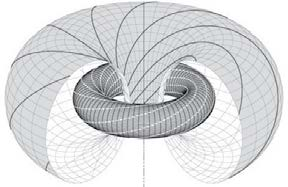## Research faculty members in this area

#### Algebraic geometry

A circle in a plane is a simple example of an algebraic curve because it has a polynomial equation in the variables x and y. In general, if we are given several such equations in a multidimensional space, then the solution set is called an algebraic variety. This is the basic object of study in Algebraic Geometry.

This is a very rich subject with connections to almost every other area of mathematics. On the pure side, it involves deep questions about the topology, intersection theory and the arithmetic of varieties. On the applied side, it is useful in cryptography, control theory, computer science and even statistics. It is a fascinating and inexhaustible field of study in which much remains to be learned and discovered.

The invariant theory is a sub-area of Algebraic Geometry, where the algebraic variety admits a group of symmetries. (For example, the circle has an obvious rotational symmetry, but in general, more complicated groups can come into play.) This ties in with several intriguing questions about the corresponding rings of invariants which can be solved using computer algebra.#### Low-dimensional topology

Low-dimensional topology is the study of phenomena that are specific to manifolds of dimension four or fewer. One approach to problems in this field is to translate, whenever possible, the available information into the language of groups. The most common method of doing this is via the “fundamental group” of a space, which encodes a remarkable amount of topological information about a space and its structure. There are also other ways of connecting group theory to the world of low-dimensional topology, for example via braid groups, covering spaces and certain topological groups.

In the broadest sense, the goal is then to become adept at translating algebraic information about the groups at hand into topological information about their corresponding spaces, and vice versa. In particular, one can study the actions of a group on spaces such as the circle, real line or a tree, and depending on the group these actions (or even the existence of such an action) can carry an incredible amount of information about the space. The exploration of these connections has been the driving force in recent years behind a reemergence of the study of orderable groups, and a new group-theoretic perspective on subtle questions relating to codimension one foliations.#### Moduli spaces of riemann surfaces

A Riemann surface is a complex two-dimensional surface; a moduli space of Riemann surfaces is a parametric family of Riemann surfaces.

Riemann surfaces appear naturally in geometry and topology and play an important role for example in complex analysis, algebraic geometry, and mathematical physics.

There are interesting interactions between an (infinite-dimensional) moduli space called Teichmuller space, and a branch of mathematical physics called conformal field theory. Conformal field theory studies quantum or statistical systems which are invariant under local rescaling and rotations. In the last few decades, it has been the focus of a great deal of interest by mathematicians because of its rich structure and deep connections to diverse fields. Mathematical and physical models of conformal field theory involve moduli spaces of Riemann surfaces.#### Symplectic geometry

Symplectic geometry is a branch of differential geometry that has origins in the mathematical framework of classical mechanics (e.g. such as Newton’s laws of motion). The main objects of study are symplectic manifolds—generalizations of Euclidean space that allow for non-trivial curvature and topology—that play the role of classical phase space (the parameter space of position and momentum coordinates).

The notion of symmetry plays an important role in symplectic geometry, and there is great interest in understanding the properties of symplectic manifolds that can detect and distinguish various kinds of symmetry. In the classical viewpoint, symmetry is manifested by an underlying group, whereby every symmetry is viewed as an invertible transformation and one can compose/multiply any two symmetries. A modern perspective on symmetry is through a generalization called a groupoid, where the composition/multiplication law is only defined for certain pairs of ‘compatible’ symmetries. Groupoid symmetries provide a robust framework in differential geometry that can handle many interesting ‘singular’ (i.e. non-manifold) spaces of interest and have permeated current research at the interface of geometry, topology and mathematical physics.